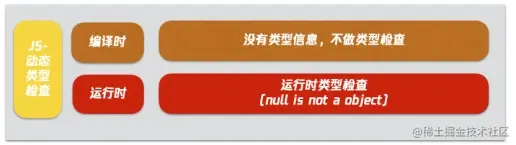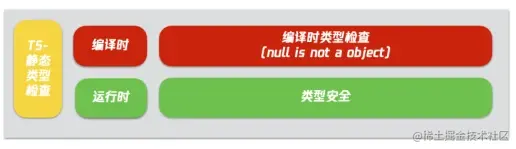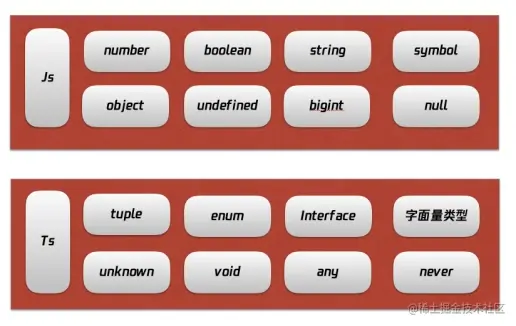# 类型体操的9种类型运算、4种类型套路总结1. 类型体操的背景，通过背景了解为什么要在项目中加入类型体操；
2. 了解类型体操的主要类型、运算逻辑、和类型套路；
3. 类型体操实践，解析 TypeScript 内置高级类型，手写 `ParseQueryString` 复杂类型；
4. 小结，综上分享，沉淀结论。

## 一、背景

### 1. 什么是类型？

• 不同类型变量占据的内存大小不同

boolean 类型的变量会分配 4 个字节的内存，而 number 类型的变量则会分配 8 个字节的内存，给变量声明了不同的类型就代表了会占据不同的内存空间。

• 不同类型变量可做的操作不同

number 类型可以做加减乘除等运算，boolean 就不可以，复合类型中不同类型的对象可用的方法不同，比如 Date 和 RegExp，变量的类型不同代表可以对该变量做的操作就不同。

### 3. 怎么实现类型安全？

#### 3.1 动态类型检查

Javascript 就是典型的动态类型检查，它在编译时，没有类型信息，到运行时才检查，导致很多隐藏 bug。#### 3.2 静态类型检查

TypeScript 作为 Javascript 的超集，采用的是静态类型检查，在编译时就有类型信息，检查类型问题，减少运行时的潜在问题。### 4. 什么是类型体操

#### 4.1 简单类型系统

``````int add(int a, int b) {
return a + b
}

double add(double a, double b) {
return a + b
}

#### 4.2 泛型类型系统

``````T add<T>(T a, T b) {
return a + b
}

``````function getPropValue<T>(obj: T, key) {
return obj[key]
}

#### 4.3 类型编程系统

``````function getPropValue<
T extends object,
Key extends keyof T
>(obj: T, key: Key): T[Key] {
return obj[key]
}

## 二、了解类型体操

### 1. 有哪些类型``````// 元组（Tuple）就是元素个数和类型固定的数组类型
type Tuple = [number, string];

// 接口（Interface）可以描述函数、对象、构造器的结构：
interface IPerson {
name: string;
age: number;
}

class Person implements IPerson {
name: string;
age: number;
}

const obj: IPerson = {
name: 'aa',
age: 18
}

// 枚举（Enum）是一系列值的复合：
enum Transpiler {
Babel = 'babel',
Postcss = 'postcss',
Terser = 'terser',
Prettier = 'prettier',
TypeScriptCompiler = 'tsc'
}

const transpiler = Transpiler.TypeScriptCompiler;

### 2. 运算逻辑

TypeScript 支持条件、推导、联合、交叉、对联合类型做映射等 9 种运算逻辑。

• 条件：T extends U ? X : Y

``````// 条件：extends ? :
// 如果 T 是 2 的子类型，那么类型是 true，否则类型是 false。
type isTwo<T> = T extends 2 ? true : false;
// false
type res = isTwo<1>;

• 约束：extends

``````// 通过 T extends Length 约束了 T 的类型，必须是包含 length 属性，且 length 的类型必须是 number。
interface Length {
length: number
}

function fn1<T extends Length>(arg: T): number{
return arg.length
}

• 推导：infer

``````// 推导：infer
// 提取元组类型的第一个元素：
// extends 约束类型参数只能是数组类型，因为不知道数组元素的具体类型，所以用 unknown。
// extends 判断类型参数 T 是不是 [infer F, ...infer R] 的子类型，如果是就返回 F 变量，如果不是就不返回
type First<T extends unknown[]> = T extends [infer F, ...infer R] ? F : never;
// 1
type res2 = First<[1, 2, 3]>;

• 联合：|

``````type Union = 1 | 2 | 3

• 交叉：&

``````type ObjType = { a: number } & { c: boolean }

• 索引查询：keyof T

keyof 用于获取某种类型的所有键，其返回值是联合类型。

``````// const a: 'name' | 'age' = 'name'
const a: keyof {
name: string,
age: number
} = 'name'

• 索引访问：T[K]

T[K] 用于访问索引，得到索引对应的值的联合类型。

``````interface I3 {
name: string,
age: number
}

type T6 = I3[keyof I3] // string | number

• 索引遍历： in

in 用于遍历联合类型。

``````const obj = {
name: 'tj',
age: 11
}

type T5 = {
[P in keyof typeof obj]: any
}

/*
{
name: any,
age: any
}
*/

• 索引重映射： as

as 用于修改映射类型的 key。

``````// 通过索引查询 keyof，索引访问 t[k]，索引遍历 in，索引重映射 as，返回全新的 key、value 构成的新的映射类型
type MapType<T> = {
[
Key in keyof T
as `\${Key & string}\${Key & string}\${Key & string}`
]: [T[Key], T[Key], T[Key]]
}
// {
//     aaa: [1, 1, 1];
//     bbb: [2, 2, 2];
// }
type res3 = MapType<{ a: 1, b: 2 }>

### 3. 运算套路

• 模式匹配做提取；
• 重新构造做变换；
• 递归复用做循环；
• 数组长度做计数。

#### 3.1 模式匹配做提取

``````type GetParameters<Func extends Function> =
Func extends (...args: infer Args) => unknown ? Args : never;

type ParametersResult = GetParameters<(name: string, age: number) => string>

#### 3.2 重新构造做变换

``````type CapitalizeStr<Str extends string> =
Str extends `\${infer First}\${infer Rest}`
? `\${Uppercase<First>}\${Rest}` : Str;

type CapitalizeResult = CapitalizeStr<'tang'>

#### 3.3 递归复用做循环

TypeScript 本身不支持循环，但是可以通过递归完成不确定数量的类型编程，达到循环的效果。

``````type ReverseArr<Arr extends unknown[]> =
Arr extends [infer First, ...infer Rest]
? [...ReverseArr<Rest>, First]
: Arr;

type ReverseArrResult = ReverseArr<[1, 2, 3, 4, 5]>

#### 3.4 数组长度做计数

``````type BuildArray<
Length extends number,
Ele = unknown,
Arr extends unknown[] = []
> = Arr['length'] extends Length
? Arr
: BuildArray<Length, Ele, [...Arr, Ele]>;

type Add<Num1 extends number, Num2 extends number> =
[...BuildArray<Num1>, ...BuildArray<Num2>]['length'];

## 三、类型体操实践

### 1. 解析 TypeScript 内置高级类型

• partial 把索引变为可选

``````type TPartial<T> = {
[P in keyof T]?: T[P];
};

type PartialRes = TPartial<{ name: 'aa', age: 18 }>

• Required 把索引变为必选

``````type TRequired<T> = {
[P in keyof T]-?: T[P]
}

type RequiredRes = TRequired<{ name?: 'aa', age?: 18 }>

``````type TReadonly<T> = {
readonly [P in keyof T]: T[P]
}

• Pick 保留过滤索引

``````type TPick<T, K extends keyof T> = {
[P in K]: T[P]
}

type PickRes = TPick<{ name?: 'aa', age?: 18 }, 'name'>

• Record 创建映射类型

``````type TRecord<K extends keyof any, T> = {
[P in K]: T
}

type RecordRes = TRecord<'aa' | 'bb', string>

• Exclude 删除联合类型的一部分

``````type TExclude<T, U> = T extends U ? never : T

type ExcludeRes = TExclude<'aa' | 'bb', 'aa'>

• Extract 保留联合类型的一部分

``````type TExtract<T, U> = T extends U ? T : never

type ExtractRes = TExtract<'aa' | 'bb', 'aa'>

• Omit 删除过滤索引

``````type TOmit<T, K extends keyof T> = Pick<T, Exclude<keyof T, K>>

type OmitRes = TOmit<{ name: 'aa', age: 18 }, 'name'>

• Awaited 用于获取 Promise 的 valueType

``````type TAwaited<T> =
T extends null | undefined
? T
: T extends object & { then(onfulfilled: infer F): any }
? F extends ((value: infer V, ...args: any) => any)
? Awaited<V>
: never
: T;

type AwaitedRes = TAwaited<Promise<Promise<Promise<string>>>>

### 2. 解析 ParseQueryString 复杂类型

``````function parseQueryString1(queryStr) {
if (!queryStr || !queryStr.length) {
return {}
}
const queryObj = {}
const items = queryStr.split('&')
items.forEach((item) => {
const [key, value] = item.split('=')
if (queryObj[key]) {
if (Array.isArray(queryObj[key])) {
queryObj[key].push(value)
} else {
queryObj[key] = [queryObj[key], value]
}
} else {
queryObj[key] = value
}
})
return queryObj
}

``````function parseQueryString1(queryStr: string): Record<string, any> {
if (!queryStr || !queryStr.length) {
return {}
}
const queryObj = {}
const items = queryStr.split('&')
items.forEach((item) => {
const [key, value] = item.split('=')
if (queryObj[key]) {
if (Array.isArray(queryObj[key])) {
queryObj[key].push(value)
} else {
queryObj[key] = [queryObj[key], value]
}
} else {
queryObj[key] = value
}
})
return queryObj
}

``````
type ParseParam<Param extends string> =
Param extends `\${infer Key}=\${infer Value}`
? {
[K in Key]: Value
} : Record<string, any>;

type MergeParams<
OneParam extends Record<string, any>,
OtherParam extends Record<string, any>
> = {
readonly [Key in keyof OneParam | keyof OtherParam]:
Key extends keyof OneParam
? OneParam[Key]
: Key extends keyof OtherParam
? OtherParam[Key]
: never
}

type ParseQueryString<Str extends string> =
Str extends `\${infer Param}&\${infer Rest}`
? MergeParams<ParseParam<Param>, ParseQueryString<Rest>>
: ParseParam<Str>;

``````function parseQueryString<Str extends string>(queryStr: Str): ParseQueryString<Str> {
if (!queryStr || !queryStr.length) {
return {} as any;
}
const queryObj = {} as any;
const items = queryStr.split('&');
items.forEach(item => {
const [key, value] = item.split('=');
if (queryObj[key]) {
if(Array.isArray(queryObj[key])) {
queryObj[key].push(value);
} else {
queryObj[key] = [queryObj[key], value]
}
} else {
queryObj[key] = value;
}
});
return queryObj as any;
}

const res = parseQueryString('a=1&b=2&c=3');

console.log(res.a) // type 1

## 四、小结

• 第一点是类型体操背景，了解了什么是类型，什么是类型安全，怎么实现类型安全；

• 第二点是熟悉类型体操的主要类型、支持的逻辑运算，并总结了 4 个类型套路；

• 第三点是类型体操实践，解析了 TypeScript 内置高级类型的实现，并手写了一些复杂函数类型。